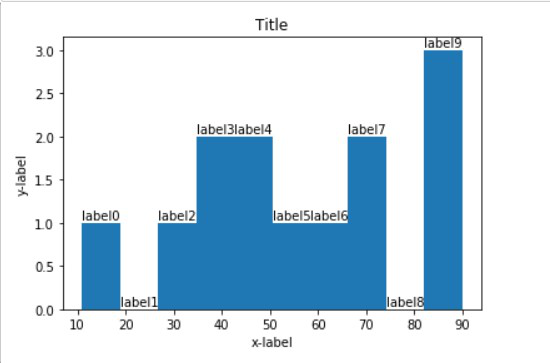Open In App

# Adding labels to histogram bars in Matplotlib

In this article, we are going to discuss how to add labels to histogram bars in matplotlib. Histograms are used to display continuous data using bars. It looks similar to the bar graph. It shows the count or frequency of element that falls under the category mentioned in that range it means, taller the graph, higher the frequency of that range. To display the histogram and its labels we are going to use matplotlib.

Approach:

• We import matplotlib and numpy library.
• Create a dataset using numpy library so that we can plot it.
• Create a histogram using matplotlib library.
• To give labels use set_xlabel() and set_ylabel() functions.
• We add label to each bar in histogram and for that, we loop over each bar and use text() function to add text over it. We also calculate height and width of each bar so that our label don’t coincide with each other.
• Use show() function to display the histogram.

Below is the code Implementation:

## Python

 `from` `matplotlib ``import` `pyplot as plt``import` `numpy as np`` ` `# Creating dataset``marks ``=` `np.array([``70``, ``50``, ``40``, ``90``, ``55``, ``85``, ``74``, ``66``, ``33``, ``11``, ``45``, ``36``, ``89``])`` ` `# Creating histogram``fig, ax ``=` `plt.subplots(``1``, ``1``)``ax.hist(marks)`` ` `# Set title``ax.set_title(``"Title"``)`` ` `# adding labels``ax.set_xlabel(``'x-label'``)``ax.set_ylabel(``'y-label'``)`` ` `# Make some labels.``rects ``=` `ax.patches``labels ``=` `[``"label%d"` `%` `i ``for` `i ``in` `range``(``len``(rects))]`` ` `for` `rect, label ``in` `zip``(rects, labels):``    ``height ``=` `rect.get_height()``    ``ax.text(rect.get_x() ``+` `rect.get_width() ``/` `2``, height``+``0.01``, label,``            ``ha``=``'center'``, va``=``'bottom'``)`` ` `# Show plot``plt.show()`

Output:Explanation:

In the above code first, we created an array using np.array(). After that, we created a histogram using hist() function. To give labels we used  set_xlabel() and set_ylabel() functions. To give title to our graph we used the set_title() function.  We also added labels to each bar and for that first we used the get_height function to get height then, we used for loop to loop over each bar and add text over it using text() function. We used label variable to store the names of variables. Finally, to display histogram we used show() function.